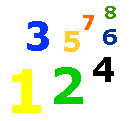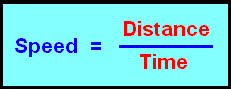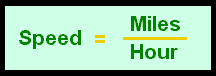# 4-15 Concept of Speed

 Speed is how fast an object travels in a unit of time.  If you travel in a car, you travel at a speed of miles per hour (mph).  If you travel in your car at 50 mph for one hour, you will have traveled 50 miles.  If you travel in your car at 50 mph for 30 minutes, you will have traveled 25 miles. Speed is expressed as units of length and time.  Length is miles and time is hours.  The "per" in miles per hour means to divide.  Thus miles divided by hours.  Look at the formula below.OrIf a truck travels 120 miles in 2 hours, its speed would be 120 divided by 2 equals 60 or 60 mph. Complete the questions below.   Speed is how fast and object travels over . Per means to . Solve.  A car travels 360 miles in 3 hours.  What is the speed of the car? mph Solve.  A truck travels 900 miles in 12 hours.  What is the speed of the truck? mph An airplane travels 3600 miles in 4 hours.  What is the speed of the airplane? mph An object travels at 300 mph for 12 hours.  What distance did the object travel? miles